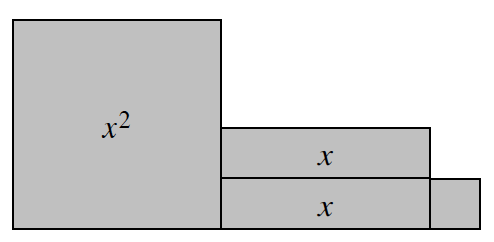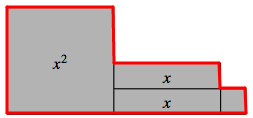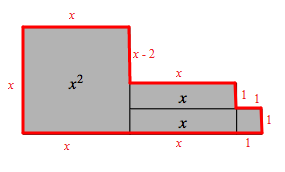### Home > CC2MN > Chapter 6 Unit 6A > Lesson CC3: 6.1.2 > Problem6-15

6-15.Write expressions for the perimeter and the area of this algebra tile shape. Then simplify each expression by combining like terms.

The perimeter is the distance around a shape.

Label the values of all of the sides.

Add these values together to find the expression for the perimeter.
$x+x+(x-2)+x+1+1+1+1+x+x$
Simplify this expression by combining like terms.

$P=6x+2$

The area is the space bound within the perimeter.

Add the areas of all $4$ tiles within the perimeter.
$x^2+x+x+1$
Now simplify this expression.